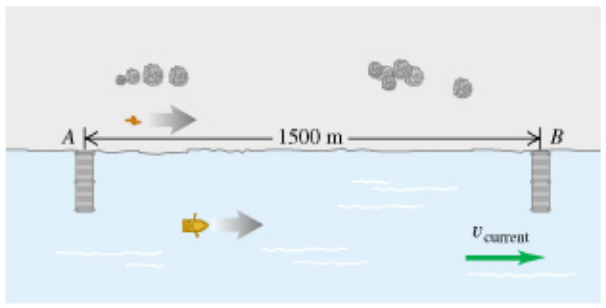# Problem: Two piers, A and B, are located on a river: B is 1500 m downstream from A. Two friends must make round trips from pier A to pier B and return. One rows a boat at a constant speed of 4.00 km/h relative to the water; the other walks on the shore at a constant speed of 4.00 km/h. The velocity of the river is 2.80 km/h in the direction from A to B.(a) How much time does it take the walker to make the round trip?(b) How much time does it take the rower to make the round trip?

###### FREE Expert Solution
93% (268 ratings)
###### FREE Expert Solution

For one-dimensional relative motion problems like this one, we’ll generally use the formula

$\overline{){{\mathbf{v}}}_{\mathbf{P}\mathbf{A}}{\mathbf{=}}{{\mathbf{v}}}_{\mathbf{P}\mathbf{B}}{\mathbf{+}}{{\mathbf{v}}}_{\mathbf{B}\mathbf{A}}}$

For example, if a boat is being propelled along a river, the boat’s velocity relative to the ground is the boat’s velocity relative to the river plus the river’s velocity relative to the ground. It’s important to keep track of the signs, so make sure you clearly define a coordinate system!

Because the velocities are constant, we'll end up using the equation

$\overline{){\mathbf{x}}{\mathbf{=}}{\mathbf{v}}{\mathbf{t}}}$

In this problem, we’re asked to calculate both the time it takes someone to walk the distance between the two piers twice at a constant speed, and the time it takes someone to row that distance once along with the current and once against the current. We’re given the walking speed, vw, which is relative to the ground; the speed of the boat relative to the water, vbr; and the speed of the river relative to the ground, vrg.

93% (268 ratings)###### Problem Details

Two piers, A and B, are located on a river: B is 1500 m downstream from A. Two friends must make round trips from pier A to pier B and return. One rows a boat at a constant speed of 4.00 km/h relative to the water; the other walks on the shore at a constant speed of 4.00 km/h. The velocity of the river is 2.80 km/h in the direction from A to B.
(a) How much time does it take the walker to make the round trip?
(b) How much time does it take the rower to make the round trip?